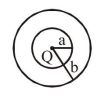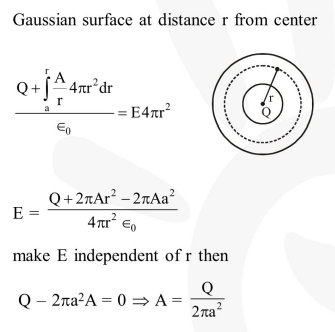Deepak Scored 45->99%ile with Bounce Back Crack Course. You can do it too!

# The region between two concentric spheres of radii 'a' and 'b',

Question:

The region between two concentric spheres of radii 'a' and 'b', respectively (see figure), has volume charge density $\rho=\frac{\mathrm{A}}{\mathrm{r}}$, where $\mathrm{A}$ is a constant and $\mathrm{r}$ is the distance from the centre. At the centre of the spheres is a point charge $Q$. The value of A such that the electric field in the region between the spheres will be constant, is :-1. $\frac{2 Q}{\pi a^{2}}$

2. $\frac{\mathrm{Q}}{2 \pi \mathrm{a}^{2}}$

3. $\frac{Q}{2 \pi\left(b^{2}-a^{2}\right)}$

4. $\frac{2 Q}{\pi\left(a^{2}-b^{2}\right)}$

Correct Option: , 2

Solution: